Pie Chart - Drawing

Chapter 4 Class 8 Data Handling
Concept wise

Suppose we are given data like

 Company Percentage of people Samsung 40% Apple 25% Vivo 20% Mi 15%

We convert

Percentage → Angles

We know that

Angle at center = 360°

So, we convert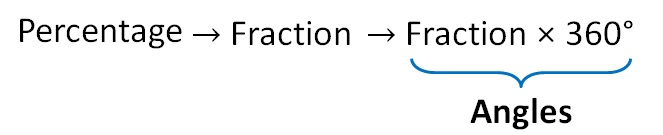So, our table becomes.

 Company Percentage of people Fraction Angle = Fraction × 360 ° Samsung 40% 40/100 = 4/10 4/10 × 360 = 4 × 36 = 144° Apple 25% 25/100 = 1/4 1/4 × 360  = 90° Vivo 20% 20/100 = 2/10 2/10 × 360 = 2 × 36 = 72° Mi 15% 15/100 = 3/20 3/20 × 360 = 3 × 18 = 54°

1. Draw a circle of any radius.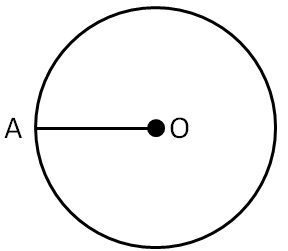1. Put protractor on AO and

Draw largest angle 144°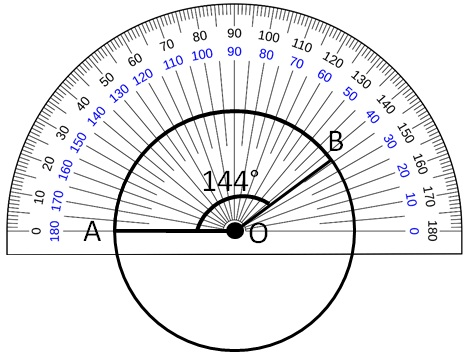1. Now, put protractor on AO

and

Mark next largest angle 90°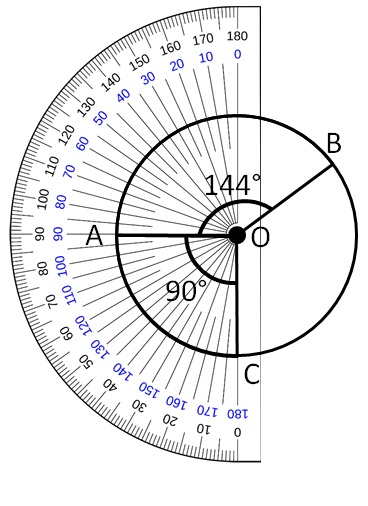1. Now, put protractor on OC and

Mark next largest angle 72°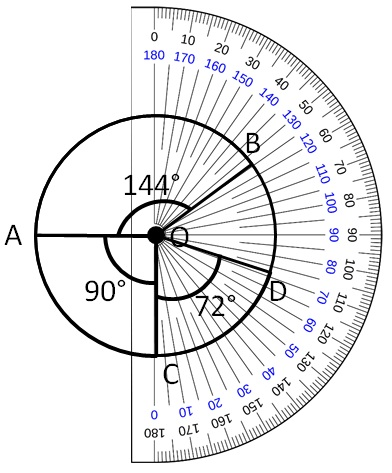1. The remaining portion is 54°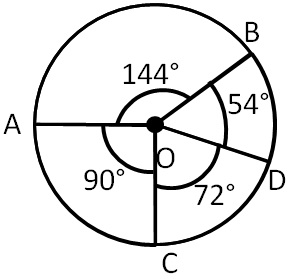1. Now, label the pie chart

 Company Percentage of people Angle Samsung 40% 144° Apple 25% 90° Vivo 20% 72° Mi 15% 54°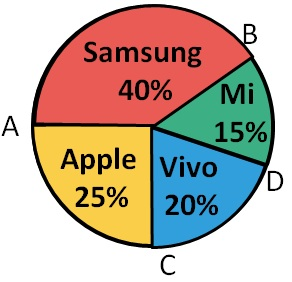Let’s take another example

## We are given the type of movie that students of class VIII like are

 Company Percentage of people Super hero 50% Animated 25% Comedy 20% Romance 5%

## Draw a pie chart of the same

Now to make pie chart we have find angles

 Company Percentage of people Fraction Angle = Fraction × 360 ° Super hero 50% 50/100 = 1/2 1/2 × 360 = 180° Animated 25% 25/100 = 1/4 1/4 × 360 = 90° Comedy 20% 20/100 = 1/5 1/5 × 360 = 72° Romance 5% 5/100 = 1/20 1/20 × 360 = 18°

1. Draw a circle of any radius.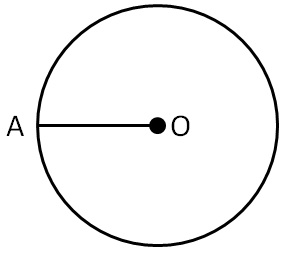1. Put protractor on OA and

Draw largest angle i.e 180°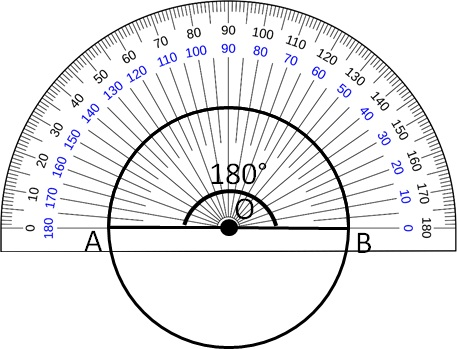1. Now, put protector on OB, and draw the next largest angle i.e 90°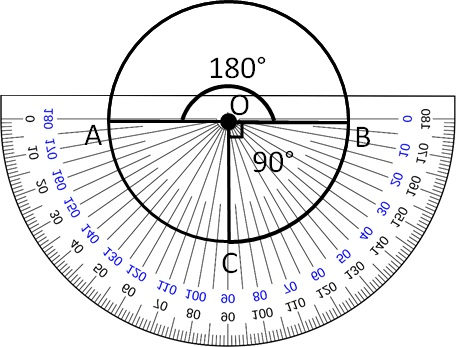1. Put protractor on OC and mark next largest angle i.e 72°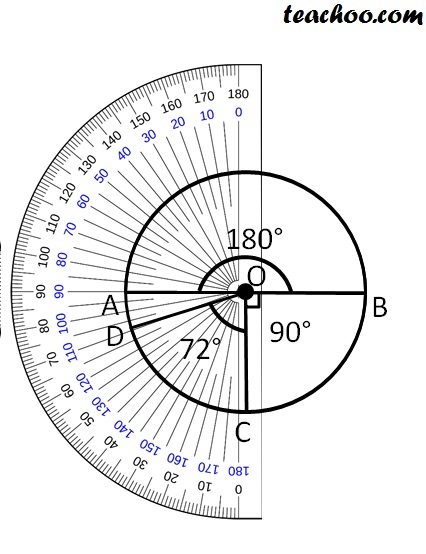1. The remaining portion is 18°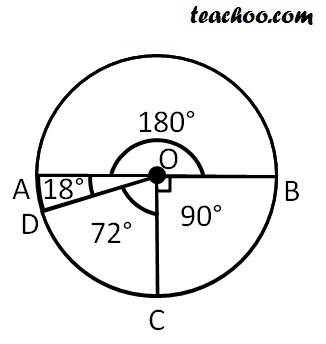1. Now, Label the pie chart
 Company Percentage of people Angle Super hero 50% 180° Animated 25% 90° Comedy 20% 72° Romance 5% 18°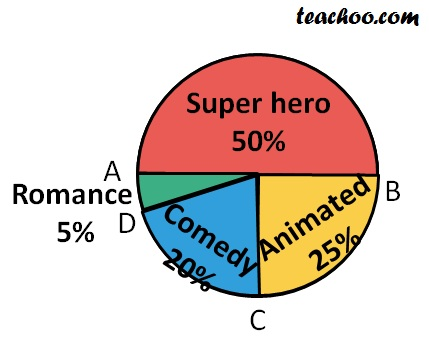Learn in your speed, with individual attention - Teachoo Maths 1-on-1 Class

### Transcript

Drawing a pie chartSuppose we are given data like We convert Percentage → Angles We know that Angle at center = 360° So, we convert So, our table becomes. We follow the steps 1. Draw a circle of any radius. Mark Radius as OA. 2. Put protractor on AO and Draw largest angle 144° 3. Now, put protractor on AO and Mark next largest angle 90° 4. Now, put protractor on OC and Mark next largest angle 72° 5. The remaining portion is 54° 6. Now, label the pie chart Let’s take another example We are given the type of movie that students of class VIII like are Draw a pie chart of the same Suppose we are given data in following from Now to make pie chart we have find angles We follow these steps 1. Draw a circle of any radius. Mark Radius as OA. 2. Put protractor on OA and Draw largest angle i.e 180° 3. Now, put protector on OB, and draw the next largest angle i.e 90° 4. Put protractor on OC and mark next largest angle i.e 72° 5. The remaining portion is 18° 6. Now, Label the pie chart